CBSE (Science) Class 11CBSE
Share

# H.C. Verma solutions for Class 12 Physics chapter 2 - Kinetic Theory of Gases

## Concepts of Physics - Vol. 2

#### H.C. Verma Concepts of Physics - Vol. 2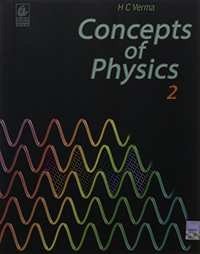## Chapter 2: Kinetic Theory of Gases

#### Chapter 2: Kinetic Theory of Gases Exercise Short Answers solutions [Page 32]

Short Answers | Q 1 | Page 32

When we place a gas cylinder on a van and the van moves, does the kinetic energy of the molecules increase? Does the temperature increase?

Short Answers | Q 2 | Page 32

While gas from a cooking gas cylinder is used, the pressure does not fall appreciably till the last few minutes. Why?

Short Answers | Q 3 | Page 32

Do you expect the gas in a cooking gas cylinder to obey the ideal gas equation?

Short Answers | Q 4 | Page 32

Can we define the temperature of (a) vacuum, (b) a single molecule?

Short Answers | Q 5 | Page 32

Comment on the following statement: the temperature of all the molecules in a sample of a gas is the same.

Short Answers | Q 6 | Page 32

Consider a gas of neutrons. Do you expect it to behave much better as an ideal gas as compared to hydrogen gas at the same pressure and temperature?

Short Answers | Q 7 | Page 32

A gas is kept in a rigid cubical container. If a load of 10 kg is put on the top of the container, does the pressure increase?

Short Answers | Q 8 | Page 32

If it were possible for a gas in a container to reach the temperature 0 K, its pressure would be zero. Would the molecules not collide with the walls? Would they not transfer momentum to the walls?

Short Answers | Q 9 | Page 32

It is said that the assumptions of kinetic theory are good for gases having low densities. Suppose a container is so evacuated that only one molecule is left in it. Which of the assumptions of kinetic theory will not be valid for such a situation? Can we assign a temperature to this gas?

Short Answers | Q 10 | Page 32

A gas is kept in an enclosure. The pressure of the gas is reduced by pumping out some gas. Will the temperature of the gas decrease by Charles's low?

Short Answers | Q 11 | Page 32

Explain why cooking is faster in a pressure cooker.

Short Answers | Q 12 | Page 32

If the molecules were not allowed to collide among themselves, would you expect more evaporation or less evaporation?

Short Answers | Q 13 | Page 32

Is it possible to boil water at room temperature, say 30°C? If we touch a flask containing water boiling at this temperature, will it be hot?

Short Answers | Q 14 | Page 32

When you come out of a river after a dip, you feel cold. Explain.

#### Chapter 2: Kinetic Theory of Gases Exercise MCQ solutions [Page 33]

MCQ | Q 1 | Page 33

Which of the following parameters is the same for molecules of all gases at a given temperature?

• Mass

• Speed

• Momentum

• Kinetic energy.

MCQ | Q 2 | Page 33

A gas behaves more closely as an ideal gas at

•  low pressure and low temperature

• low pressure and high temperature

• high pressure and low temperature

• high pressure and high temperature.

MCQ | Q 3 | Page 33

The pressure of an ideal gas is written as $P = \frac{2E}{3V}$ . Here E refers to

• translational kinetic energy

• rotational kinetic energy

• vibrational kinetic energy

• total kinetic energy.

MCQ | Q 4 | Page 33

The energy of a given sample of an ideal gas depends only on its

• volume

• pressure

• density

• temperature

MCQ | Q 5 | Page 33

Which of the following gases has maximum rms speed at a given temperature?

• hydrogen

• nitrogen

• oxygen

• carbon dioxide.

MCQ | Q 6 | Page 33

Figure shows graphs of pressure vs density for an ideal gas at two temperatures T1 and T2.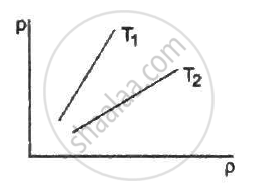• T1 > T2

• T1 = T2

• T1 < T2

• Any of the three is possible.

MCQ | Q 7 | Page 33

The mean square speed of the molecules of a gas at absolute temperature T is proportional to

• $\frac{1}{T}$

• $\sqrt{T}$

• T

• T2

MCQ | Q 8 | Page 33

Suppose a container is evacuated to leave just one molecule of a gas in it. Let va and vrms represent the average speed and the rms speed of the gas.

• va > vrms

• va < vrms

• va = vrms

• vrms is undefined.

MCQ | Q 9 | Page 33

The rms speed of oxygen at room temperature is about 500 m/s. The rms speed of hydrogen at the same temperature is about

• 125 m s−1

• 2000 m s−1

• 8000 m s−1

• 31 m s−1.

MCQ | Q 10 | Page 33

The pressure of a gas kept in an isothermal container is 200 kPa. If half the gas is removed from it, the pressure will be

• 100 kPa

• 200 kPa

• 400 kPa

• 800 kPa

MCQ | Q 11 | Page 33

The rms speed of oxygen molecules in a gas is v. If the temperature is doubled and the oxygen molecules dissociate into oxygen atoms, the rms speed will become

• v

• $v\sqrt{2}$

• 2v

•  4v

MCQ | Q 12 | Page 33

The quantity $\frac{pV}{kT}$ represents

• mass of the gas

• kinetic energy of the gas

• number of moles of the gas

• number of molecules in the gas.

MCQ | Q 13 | Page 33

The process on an ideal gas, shown in figure, is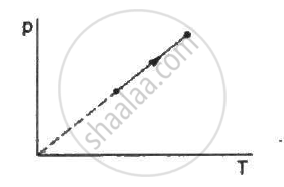• isothermal

• isobaric

• isochoric

• none of these

MCQ | Q 14 | Page 33

There is some liquid in a closed bottle. The amount of liquid is continuously decreasing. The vapour in the remaining part

• must be saturated

• must be unsaturated

• may be saturated

• there will be no vapour.

MCQ | Q 15 | Page 33

There is some liquid in a closed bottle. The amount of liquid remains constant as time passes. The vapour in the remaining part

• must be saturated

• must be unsaturated

• may be unsaturated

• there will be no vapour.

MCQ | Q 16 | Page 33

Vapour is injected at a uniform rate in a closed vessel which was initially evacuated. The pressure in the vessel

• increases continuously

• decreases continuously

• first increases and then decreases

• first increases and then becomes constant.

MCQ | Q 17 | Page 33

A vessel A has volume V and a vessel B has volume 2V. Both contain some water which has a constant volume. The pressure in the space above water is pa for vessel A and pbfor vessel B.

• pa = pb

• pa = 2pb

•  pb = 2pa

• pb = 4pa

#### Chapter 2: Kinetic Theory of Gases Exercise MCQ solutions [Pages 33 - 34]

MCQ | Q 1 | Page 33

Consider a collision between an oxygen molecule and a hydrogen molecule in a mixture of oxygen and hydrogen kept at room temperature. Which of the following are possible?
(a) The kinetic energies of both the molecules increase.
(b) The kinetic energies of both the molecules decrease.
(c) kinetic energy of the oxygen molecule increases and that of the hydrogen molecule decreases.
(d) The kinetic energy of the hydrogen molecule increases and that of the oxygen molecule decreases.

MCQ | Q 2 | Page 34

Consider a mixture of oxygen and hydrogen kept at room temperature. As compared to a hydrogen molecule an oxygen molecule hits the wall

• with greater average speed

• with smaller average speed

• with greater average kinetic energy

• with smaller average kinetic energy

MCQ | Q 3 | Page 34

Which of the following quantities is zero on an average for the molecules of an ideal gas in equilibrium?

• Kinetic energy

• Momentum

• Density

• Speed

MCQ | Q 4 | Page 34

Keeping the number of moles, volume and temperature the same, which of the following are the same for all ideal gases?

• Rms speed of a molecule

• Density

• Pressure

• Average magnitude of momentum.

MCQ | Q 5 | Page 34

The average momentum of a molecule in a sample of an ideal gas depends on

• temperature

• number of moles

• volume

• none of these.

MCQ | Q 6 | Page 34

Which of the following quantities is the same for all ideal gases at the same temperature?
(a) The kinetic energy of 1 mole
(b) The kinetic energy of 1 g
(c) The number of molecules in 1 mole
(d) The number of molecules in 1 g

MCQ | Q 7 | Page 34

Consider the quantity $\frac{MkT}{pV}$ of an ideal gas where M is the mass of the gas. It depends on the

• temperature of the gas

• volume of the gas

• pressure of the gas

• nature of the gas.

#### Chapter 2: Kinetic Theory of Gases solutions [Pages 34 - 37]

Q 1 | Page 34

Calculate the volume of 1 mole of an ideal gas at STP.

Q 2 | Page 34

Find the number of molecules of an ideal gas in a volume of 1.000 cm3 at STP.

Q 3 | Page 34

Find the number of molecules in 1 cm3 of an ideal gas at 0°C and at a pressure of 10−5mm of mercury.

Use R = 8.31 J K-1 mol-1

Q 4 | Page 34

Calculate the mass of 1 cm3 of oxygen kept at STP.

Q 5 | Page 34

Equal masses of air are sealed in two vessels, one of volume V0 and the other of volume 2V0. If the first vessel is maintained at a temperature 300 K and the other at 600 K, find the ratio of the pressures in the two vessels.

Use R = 8.31 JK-1 mol-1

Q 6 | Page 34

An electric bulb of volume 250 cc was sealed during manufacturing at a pressure of 10−3 mm of mercury at 27°C. Compute the number of air molecules contained in the bulb. Avogadro constant = 6 × 1023 mol−1, density of mercury = 13600 kg m−3 and g = 10 m s−2.

Use R=8.314J K-1 mol-1

Q 7 | Page 34

A gas cylinder has walls that can bear a maximum pressure of 1.0 × 106 Pa. It contains a gas at 8.0 × 105 Pa and 300 K. The cylinder is steadily heated. Neglecting any change in the volume, calculate the temperature at which the cylinder will break.

Q 8 | Page 34

2 g of hydrogen is sealed in a vessel of volume 0.02 m3 and is maintained at 300 K. Calculate the pressure in the vessel.

Use R=8.3J K-1 mol-1

Q 9 | Page 34

The density of an ideal gas is 1.25 × 10−3 g cm−3 at STP. Calculate the molecular weight of the gas.

Use R=8.31J K-1 mol-1

Q 10 | Page 34

The temperature and pressure at Simla are 15.0°C and 72.0 cm of mercury and at Kalka these are 35.0°C and 76.0 cm of mercury. Find the ratio of air density at Kalka to the air density at Simla.

Use R=8.314J K-1 mol-1

Q 11 | Page 34

Figure shows a cylindrical tube with adiabatic walls and fitted with a diathermic separator. The separator can be slid in the tube by an external mechanism. An ideal gas is injected into the two sides at equal pressures and equal temperatures. The separator remains in equilibrium at the middle. It is now slid to a position where it divides the tube in the ratio of 1:3. Find the ratio of the pressures in the two parts of the vessel.

Use R=8.314J K-1 mol-1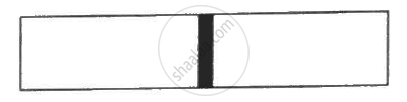Q 12 | Page 34

Find the rms speed of hydrogen molecules in a sample of hydrogen gas at 300 K. Find the temperature at which the rms speed is double the speed calculated in the previous part.

Use R=8.314 JK-1 mol-1

Q 13 | Page 34

A sample of 0.177 g of an ideal gas occupies 1000 cm3 at STP. Calculate the rms speed of the gas molecules.

Q 14 | Page 34

The average translational kinetic energy of air molecules is 0.040 eV (1 eV = 1.6 × 10−19J). Calculate the temperature of the air. Boltzmann constant k = 1.38 × 10−23 J K−1.

Q 15 | Page 34

Consider a sample of oxygen at 300 K. Find the average time taken by a molecule to travel a distance equal to the diameter of the earth.

Use R=8.314 JK-1 mol-1

Q 16 | Page 34

Find the average magnitude of linear momentum of a helium molecule in a sample of helium gas at 0°C. Mass of a helium molecule = 6.64 × 10−27 kg and Boltzmann constant = 1.38 × 10−23 J K−1.

Q 17 | Page 34

The mean speed of the molecules of a hydrogen sample equals the mean speed of the molecules of a helium sample. Calculate the ratio of the temperature of the hydrogen sample to the temperature of the helium sample.

Use R = 8.314 JK-1 mol-1

Q 18 | Page 34

At what temperature the mean speed of the molecules of hydrogen gas equals the escape speed from the earth?

Use R = 8.314 JK-1 mol-1

Q 19 | Page 35

Find the ratio of the mean speed of hydrogen molecules to the mean speed of nitrogen molecules in a sample containing a mixture of the two gases.

Use R = 8.314 JK-1 mol-1

Q 20 | Page 35

Figure shows a vessel partitioned by a fixed diathermic separator. Different ideal gases are filled in the two parts. The rms speed of the molecules in the left part equals the mean speed of the molecules in the right part. Calculate the ratio of the mass of a molecule in the left part to the mass of a molecule in the right part.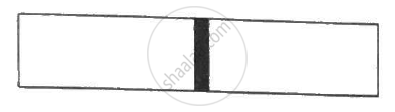Q 21 | Page 35

Estimate the number of collisions per second suffered by a molecule in a sample of hydrogen at STP. The mean free path (average distance covered by a molecule between successive collisions) = 1.38 × 10−5 cm.

Use R = 8.31 JK-1 mol-1

Q 22 | Page 35

Hydrogen gas is contained in a closed vessel at 1 atm (100 kPa) and 300 K. (a) Calculate the mean speed of the molecules. (b) Suppose the molecules strike the wall with this speed making an average angle of 45° with it. How many molecules strike each square metre of the wall per second?

Use R = 8.31 JK-1 mol-1

Q 23 | Page 35

Air is pumped into an automobile tyre's tube up to a pressure of 200 kPa in the morning when the air temperature is 20°C. During the day the temperature rises to 40°C and the tube expands by 2%. Calculate the pressure of the air in the tube at this temperature.

Q 24 | Page 35

Oxygen is filled in a closed metal jar of volume 1.0 × 10−3 m3 at a pressure of 1.5 × 105Pa and temperature 400 K. The jar has a small leak in it. The atmospheric pressure is 1.0 × 105 Pa and the atmospheric temperature is 300 K. Find the mass of the gas that leaks out by the time the pressure and the temperature inside the jar equalise with the surrounding.

Q 25 | Page 35

An air bubble of radius 2.0 mm is formed at the bottom of a 3.3 m deep river. Calculate the radius of the bubble as it comes to the surface. Atmospheric pressure = 1.0 × 105 Pa and density of water = 1000 kg m−3.

Q 26 | Page 35

Air is pumped into the tubes of a cycle rickshaw at a pressure of 2 atm. The volume of each tube at this pressure is 0.002 m3. One of the tubes gets punctured and the volume of the tube reduces to 0.0005 m3. How many moles of air have leaked out? Assume that the temperature remains constant at 300 K and that the air behaves as an ideal gas.

Use R = 8.3 J K-1 mol-1

Q 27 | Page 35

0.040 g of He is kept in a closed container initially at 100.0°C. The container is now heated. Neglecting the expansion of the container, calculate the temperature at which the internal energy is increased by 12 J.

Use R = 8.3 J K-1 mol-1

Q 28 | Page 35

During an experiment, an ideal gas is found to obey an additional law pV2 = constant. The gas is initially at a temperature T and volume V. Find the temperature when it expands to a volume 2V.

Use R = 8.3 J K-1 mol-1

Q 29 | Page 35

A vessel contains 1.60 g of oxygen and 2.80 g of nitrogen. The temperature is maintained at 300 K and the volume of the vessel is 0.166 m3. Find the pressure of the mixture.

Use R = 8.3 J K-1 mol-1

Q 30 | Page 35

A vertical cylinder of height 100 cm contains air at a constant temperature. The top is closed by a frictionless light piston. The atmospheric pressure is equal to 75 cm of mercury. Mercury is slowly poured over the piston. Find the maximum height of the mercury column that can be put on the piston.

Q 31 | Page 35

Figure shows two vessels A and B with rigid walls containing ideal gases. The pressure, temperature and the volume are pA, TA, V in the vessel A and pB, TB, V in the vessel B. The vessels are now connected through a small tube. Show that the pressure p and the temperature T satisfy Ρ/T = 1/2 ({P_A}/{T_A}+{P_B}/{T_B)) when equilibrium is achieved.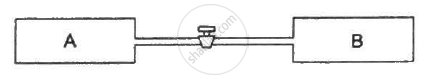Q 32 | Page 35

A container of volume 50 cc contains air (mean molecular weight = 28.8 g) and is open to atmosphere where the pressure is 100 kPa. The container is kept in a bath containing melting ice (0°C). (a) Find the mass of the air in the container when thermal equilibrium is reached. (b) The container is now placed in another bath containing boiling water (100°C). Find the mass of air in the container. (c) The container is now closed and placed in the melting-ice bath. Find the pressure of the air when thermal equilibrium is reached.

Use R = 8.3 J K-1 mol-1

Q 33 | Page 35

A uniform tube closed at one end, contains a pellet of mercury 10 cm long. When the tube is kept vertically with the closed-end upward, the length of the air column trapped is 20 cm. Find the length of the air column trapped when the tube is inverted so that the closed-end goes down. Atmospheric pressure = 75 cm of mercury.

Q 34 | Page 35

A glass tube, sealed at both ends, is 100 cm long. It lies horizontally with the middle 10 cm containing mercury. The two ends of the tube contain air at 27°C and at a pressure 76 cm of mercury. The air column on one side is maintained at 0°C and the other side is maintained at 127°C. Calculate the length of the air column on the cooler side. Neglect the changes in the volume of mercury and of the glass.

Q 35 | Page 35

An ideal gas is trapped between a mercury column and the closed-end of a narrow vertical tube of uniform base containing the column. The upper end of the tube is open to the atmosphere. The atmospheric pressure equals 76 cm of mercury. The lengths of the mercury column and the trapped air column are 20 cm and 43 cm respectively. What will be the length of the air column when the tube is tilted slowly in a vertical plane through an angle of 60°? Assume the temperature to remain constant.

Q 36 | Page 36

Figure shows a cylindrical tube of length 30 cm which is partitioned by a tight-fitting separator. The separator is very weakly conducting and can freely slide along the tube. Ideal gases are filled in the two parts of the vessel. In the beginning, the temperatures in the parts A and B are 400 K and 100 K respectively. The separator slides to a momentary equilibrium position shown in the figure. Find the final equilibrium position of the separator, reached after a long time.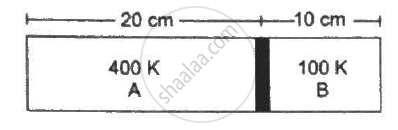Q 37 | Page 36

A vessel of volume V0 contains an ideal gas at pressure p0 and temperature T. Gas is continuously pumped out of this vessel at a constant volume-rate dV/dt = r keeping the temperature constant. The pressure of the gas being taken out equals the pressure inside the vessel. Find (a) the pressure of the gas as a function of time, (b) the time taken before half the original gas is pumped out.

Use R = 8.3 J K-1 mol-1

Q 38 | Page 36

One mole of an ideal gas undergoes a process P = (P_0)/(1+(V/V_0)^2 where p_0 and V_0 are constants . Find the temperature of the gas when V=V_0 .

Q 39 | Page 36

Show that the internal energy of the air (treated as an ideal gas) contained in a room remains constant as the temperature changes between day and night. Assume that the atmospheric pressure around remains constant and the air in the room maintains this pressure by communicating with the surrounding through the windows, doors, etc.

Use R = 8.314 J K-1 mol-1

Q 40 | Page 36

Figure shows a cylindrical tube of radius 5 cm and length 20 cm. It is closed by a tight-fitting cork. The friction coefficient between the cork and the tube is 0.20. The tube contains an ideal gas at a pressure of 1 atm and a temperature of 300 K. The tube is slowly heated and it is found that the cork pops out when the temperature reaches 600 K. Let dN denote the magnitude of the normal contact force exerted by a small length dlof the cork along the periphery (see the figure). Assuming that the temperature of the gas is uniform at any instant, calculate (dN)/(dt).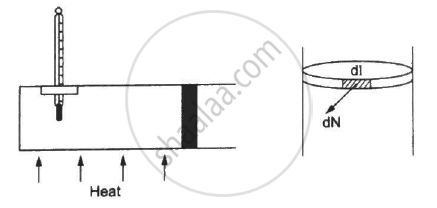Q 41 | Page 36

Figure shows a cylindrical tube of cross-sectional area A fitted with two frictionless pistons. The pistons are connected to each other by a metallic wire. Initially, the temperature of the gas is T0 and its pressure is p0 which equals the atmospheric pressure. (a) What is the tension in the wire? (b) What will be the tension if the temperature is increased to 2T0 ?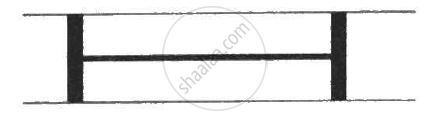Q 42 | Page 36

Figure shows a large closed cylindrical tank containing water. Initially the air trapped above the water surface has a height h0 and pressure 2p0 where p0 is the atmospheric pressure. There is a hole in the wall of the tank at a depth h1 below the top from which water comes out. A long vertical tube is connected as shown. (a) Find the height h2 of the water in the long tube above the top initially. (b) Find the speed with which water comes out of the hole. (c) Find the height of the water in the long tube above the top when the water stops coming out of the hole.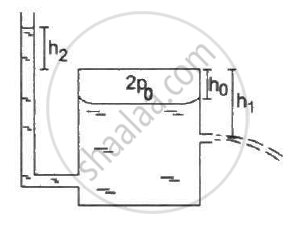Q 43 | Page 36

An ideal gas is kept in a long cylindrical vessel fitted with a frictionless piston of cross-sectional area 10 cm2 and weight 1 kg in figure. The vessel itself is kept in a big chamber containing air at atmospheric pressure 100 kPa. The length of the gas column is 20 cm. If the chamber is now completely evacuated by an exhaust pump, what will be the length of the gas column? Assume the temperature to remain constant throughout the process.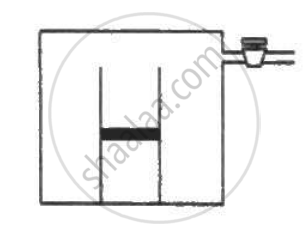Q 44 | Page 36

An ideal gas is kept in a long cylindrical vessel fitted with a frictionless piston of cross-sectional area 10 cm2 and weight 1 kg. The length of the gas column in the vessel is 20 cm. The atmospheric pressure is 100 kPa. The vessel is now taken into a spaceship revolving round the earth as a satellite. The air pressure in the spaceship is maintained at 100 kPa. Find the length of the gas column in the cylinder.

Use R = 8.3 J K-1 mol-1

Q 45 | Page 36

Two glass bulbs of equal volume are connected by a narrow tube and are filled with a gas at 0°C at a pressure of 76 cm of mercury. One of the bulbs is then placed in melting ice and the other is placed in a water bath maintained at 62°C. What is the new value of the pressure inside the bulbs? The volume of the connecting tube is negligible.

Q 46 | Page 37

The weather report reads, "Temperature 20°C : Relative humidity 100%". What is the dew point?

Q 47 | Page 37

The condition of air in a closed room is described as follows. Temperature = 25°C, relative humidity = 60%, pressure = 104 kPa. If all the water vapour is removed from the room without changing the temperature, what will be the new pressure? The saturation vapour pressure at 25°C − 3.2 kPa.

Q 48 | Page 37

The temperature and the dew point in an open room are 20°C and 10°C. If the room temperature drops to 15°C, what will be the new dew point?

Q 49 | Page 37

Pure water vapour is trapped in a vessel of volume 10 cm3. The relative humidity is 40%. The vapour is compressed slowly and isothermally. Find the volume of the vapour at which it will start condensing.

Q 50 | Page 37

A barometer tube is 80 cm long (above the mercury reservoir). It reads 76 cm on a particular day. A small amount of water is introduced in the tube and the reading drops to 75.4 cm. Find the relative humidity in the space above the mercury column if the saturation vapour pressure at the room temperature is 1.0 cm.

Q 51 | Page 37

Using figure, find the boiling point of methyl alcohol at 1 atm (760 mm of mercury) and at 0.5 atm.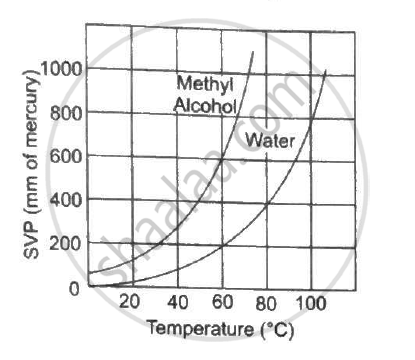Q 52 | Page 37

The human body has an average temperature of 98°F. Assume that the vapour pressure of the blood in the veins behaves like that of pure water. Find the minimum atmospheric pressure which is necessary to prevent the blood from boiling. Use figure for the vapour pressures.Q 53 | Page 37

A glass contains some water at room temperature 20°C. Refrigerated water is added to it slowly. when the temperature of the glass reaches 10°C, small droplets condense on the outer surface. Calculate the relative humidity in the room. The boiling point of water at a pressure of 17.5 mm of mercury is 20°C and at 8.9 mm of mercury it is 10°C.

Q 54 | Page 37

50 m3 of saturated vapour is cooled down from 30°C to 20°C. Find the mass of the water condensed. The absolute humidity of saturated water vapour is 30 g m−3 at 30°C and 16 g m−3 at 20°C.

Q 55 | Page 37

A barometer correctly reads the atmospheric pressure as 76 cm of mercury. Water droplets are slowly introduced into the barometer tube by a dropper. The height of the mercury column first decreases and then becomes constant. If the saturation vapour pressure at the atmospheric temperature is 0.80 cm of mercury, find the height of the mercury column when it reaches its minimum value.

Q 56 | Page 37

50 cc of oxygen is collected in an inverted gas jar over water. The atmospheric pressure is 99.4 kPa and the room temperature is 27°C. The water level in the jar is same as the level outside. The saturation vapour pressure at 27°C is 3.4 kPa. Calculate the number of moles of oxygen collected in the jar.

Q 57 | Page 37

A faulty barometer contains certain amount of air and saturated water vapour. It reads 74.0 cm when the atmospheric pressure is 76.0 cm of mercury and reads 72.10 cm when the atmospheric pressure is 74.0 cm of mercury. Saturation vapour pressure at the air temperature = 1.0 cm of mercury. Find the length of the barometer tube above the mercury level in the reservoir.

Q 58 | Page 37

On a winter day, the outside temperature is 0°C and relative humidity 40%. The air from outside comes into a room and is heated to 20°C. What is the relative humidity in the room? The saturation vapour pressure at 0°C is 4.6 mm of mercury and at 20°C it is 18 mm of mercury.

Q 59 | Page 37

The temperature and humidity of air are 27°C and 50% on a particular day. Calculate the amount of vapour that should be added to 1 cubic metre of air to saturate it. The saturation vapour pressure at 27°C = 3600 Pa.

Use R = 8.3 J K-1 mol-1

Q 60 | Page 37

The temperature and relative humidity in a room are 300 K and 20% respectively. The volume of the room is 50 m3. The saturation vapour pressure at 300 K 3.3 kPa. Calculate the mass of the water vapour present in the room.

Use R = 8.3 J K-1 mol-1

Q 61 | Page 37

The temperature and the relative humidity are 300 K and 20% in a room of volume 50 m3. The floor is washed with water, 500 g of water sticking on the floor. Assuming no communication with the surrounding, find the relative humidity when the floor dries. The changes in temperature and pressure may be neglected. Saturation vapour pressure at 300 K = 3.3 kPa.

Use R = 8.31 J K-1 mol-1

Q 62 | Page 37

A bucket full of water is placed in a room at 15°C with initial relative humidity 40%. The volume of the room is 50 m3. (a) How much water will evaporate? (b) If the room temperature is increased by 5°C, how much more water will evaporate? The saturation vapour pressure of water at 15°C and 20°C are 1.6 kPa and 2.4 kPa respectively.

Use R = 8.3 J K-1 mol-1

## Chapter 2: Kinetic Theory of Gases

#### H.C. Verma Concepts of Physics - Vol. 2## H.C. Verma solutions for Class 12 Physics chapter 2 - Kinetic Theory of Gases

H.C. Verma solutions for Class 12 Physics chapter 2 (Kinetic Theory of Gases) include all questions with solution and detail explanation. This will clear students doubts about any question and improve application skills while preparing for board exams. The detailed, step-by-step solutions will help you understand the concepts better and clear your confusions, if any. Shaalaa.com has the CBSE Concepts of Physics - Vol. 2 solutions in a manner that help students grasp basic concepts better and faster.

Further, we at Shaalaa.com provide such solutions so that students can prepare for written exams. H.C. Verma textbook solutions can be a core help for self-study and acts as a perfect self-help guidance for students.

Concepts covered in Class 12 Physics chapter 2 Kinetic Theory of Gases are Specific Heat Capacities - Gases, Mean Free Path, Kinetic Theory of Gases - Concept of Pressure, Behaviour of Gases, Equation of State of a Perfect Gas, Work Done in Compressing a Gas, Introduction of Kinetic Theory of an Ideal Gas, Kinetic Interpretation of Temperature, Kinetic Theory of Gases- Assumptions, rms Speed of Gas Molecules, Degrees of Freedom, Avogadro's Number, Molecular Nature of Matter, Law of Equipartition of Energy.

Using H.C. Verma Class 12 solutions Kinetic Theory of Gases exercise by students are an easy way to prepare for the exams, as they involve solutions arranged chapter-wise also page wise. The questions involved in H.C. Verma Solutions are important questions that can be asked in the final exam. Maximum students of CBSE Class 12 prefer H.C. Verma Textbook Solutions to score more in exam.

Get the free view of chapter 2 Kinetic Theory of Gases Class 12 extra questions for Physics and can use Shaalaa.com to keep it handy for your exam preparation

S